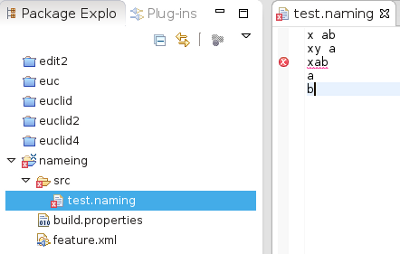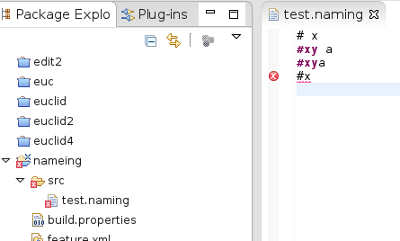# Eclipse Xtext Lexer

Lexical analysis is the process of converting a sequence of characters into a sequence of tokens.

Terminal rules, each terminal rule returns an atomic type.

Syntax Element Means
. wild card
! 'x' invert (not x)
('0'..'9') character range

In Xtext tokens are defined in the grammar file by using a terminal statement like this:

 `terminal ID : ('a'..'z'|'A'..'Z'|('_'.)) ('a'..'z'|'A'..'Z'|('_'.)|'0'..'9')*'!'?'?'?; `

A default set of terminals is provided in org.eclipse.xtext.common.Terminals:

 ``` grammar org.eclipse.xtext.common.Terminals hidden(WS, ML_COMMENT, SL_COMMENT) import "http://www.eclipse.org/emf/2002/Ecore" as ecore terminal ID : '^'?('a'..'z'|'A'..'Z'|'_')('a'..'z'|'A'..'Z'|'_'|'0'..'9')*; terminal INT returns ecore::EInt: ('0'..'9')+; terminal STRING : '"' ( '\\'('b'|'t'|'n'|'f'|'r'|'u'|'"'|"'"|'\\') | !('\\'|'"') )* '"' | "'" ( '\\'('b'|'t'|'n'|'f'|'r'|'u'|'"'|"'"|'\\') | !('\\'|"'") )* "'"; terminal ML_COMMENT : '/*' -> '*/'; terminal SL_COMMENT : '//' !('\n'|'\r')* ('\r'? '\n')?; terminal WS : (' '|'\t'|'\r'|'\n')+; terminal ANY_OTHER: .;```

We can also define 'keywords' within the main part of the parser.

So how do we decide what constructs of our DSL should be implemented as a token (terminal) and what should be implemented as a grammar rule containing many tokens?

So lets take a simple grammar file and see how tokens are handled:

## Example 1

 ```grammar com.euclideanspace.naming.Example with org.eclipse.xtext.common.Terminals generate example "https://www.euclideanspace.com/naming/Example" Model: a+=Elem*; Elem: (XY ID)|(X ID); terminal XY:'xy'; terminal X:'x';``` I then ran the mwe2 file to build the language and then ran it in a second Eclipse instance.As we can see, the program accepts x or xy followed by some other string, but only if there is a space between them.

The error message is: missing EOF at 'xab'

(no errors are detected after this error)

So would this function in the same way if we changed the terminals to ordinary keywords?

 ```grammar com.euclideanspace.naming.Example with org.eclipse.xtext.common.Terminals generate example "https://www.euclideanspace.com/naming/Example" Model: a+=Elem*; Elem: ('xy' ID)|('x' ID); ```

When regenerated and run this behaves exactly the same.

So lets step through how the lexer might work to try to guess what might be happening here:

First the tokens that I can see are:
• xy
• x
• ID
• WS (whitespace)

So lets start to parse 'xya'.

This could potentially match any of:

• 'xy'ID
• 'x'ID
• 'ID

Note at this stage we are only tokenising (lexing) not yet parsing so we are not using the parsing rules.

First we look at 'x', this could potentially match token xy , x or ID. So we can't choose yet.
 x y a WS

Next we look at 'y' here we could either:

• Issue x as a token and then start reading a new token
• Reject x as a token and continue checking for xy and ID

We can't keep both options open because lex can't backtrack, we have to choose.

Lex tries to eat longest tokens so it chooses the second option.

 x y a WS

Next we look at 'a' here we could either:

• Issue xy as a token and then start reading a new token
• Reject xy as a token and continue checking for and ID

Again lex can't backtrack an it tries to eat longest tokens so it chooses the second option.

 x y a WS
Lex gets to WS (whitespace). This does not match ID, our only remaining option, we therefore issue ID as a token.
 x y a WS

We then go on to parse the code, but no rules start with ID, so parser fails. (even though it could have successfully parsed if lex had known what parser needed).

## Example 2

 ```grammar com.euclideanspace.naming.Example with org.eclipse.xtext.common.Terminals generate example "https://www.euclideanspace.com/naming/Example" Model: a+=Elim*; Elim: Hash | HashXY; Hash: '#' ID ; HashXY: '#xy' ID;``` There seems to be something more complicated going on here. There is no need for a space after the '#xy' keyword but there is after the '#' keyword. What is going on here? Lets try stepping through the input again.First the tokens that I can see are:
• #xy
• #
• ID
• WS (whitespace)

So lets start to parse '#x'.

This could potentially match:

• '#ID'

First we look at '#', this could potentially match tokens #xy , or #. So we can't choose yet.
 # x WS

Next we look at 'x' here we could either:

• Issue # as a token and then start reading a new token
• Reject # as a token and continue checking for #xy.

We can't keep both options open because lex can't backtrack, we have to choose.

Lex tries to eat longest tokens so it chooses the second option.

 # x WS

Next we look at WS (whitespace). The only remaining option that we have open is the #xy token, but that does not match, so in this case lex fails. (even though it could have matched #ID if it had been smarter.

 # x WS

## Example 3 - Floating Point Literals

 Floating point literals seem specially difficult to implement in a grammar. Examples of floating point literals are shown on the right: 1.0 3.45e-4

So how do we implement this in a language? Does it make sense to implement this as a terminal rule?

The Xtext documentation suggests implementing floating point literals using a data type rule rather than a terminal rule (but it does not say exactly how to do it).

If we were going to implement floating point literals using terminal rules then here is a first try:
 ```terminal TK_FLOAT returns ecore::EBigDecimal: (('0'..'9')+ '.' ('0'..'9')+) (('e' | 'E') ('+' | '-')? ('0'..'9')+)?;```

The problem with a terminal rule is that it could hide other rules in a similar way to examples 1 and 2. The qualified name

However, if we don't use a terminal rule, will 'e' become a keyword? The way that Xbase seems to get round it is to implement it partly in a terminal rule but put the decimal point part in a data type rule:

The terminal rule in Xbase grammar is:
 ```terminal INT returns ecore::EInt: '0'..'9' ('0'..'9'|'_')*; terminal DECIMAL: INT (('e'|'E') ('+'|'-')? INT)? (('b'|'B')('i'|'I'|'d'|'D') | ('l'|'L'|'d'|'D'|'f'|'F'))?;```
But the terminal rule does not implement decimal point '.' so we have a data type rule to implement that part:
 ```Number hidden(): HEX | (INT | DECIMAL) ('.' (INT | DECIMAL))?; ```

### Customising Lexer

For information about customising the lexer see page here.